# Physics 2102 Jonathan Dowling Physics 2102 Lecture 10

• Slides: 13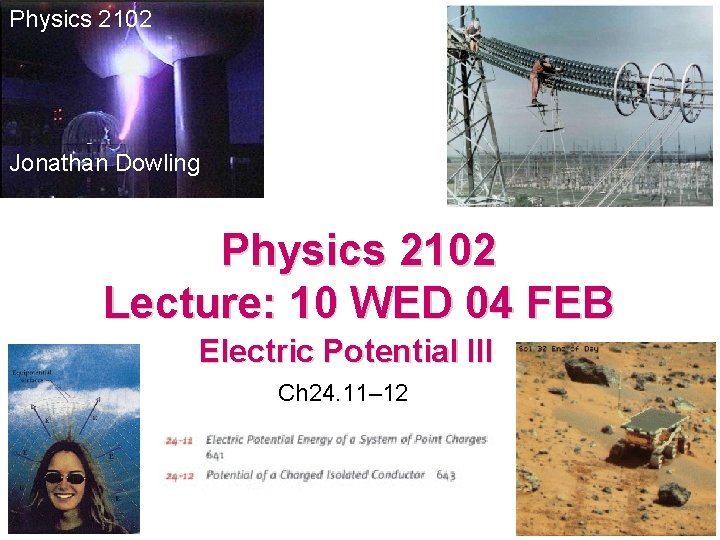Physics 2102 Jonathan Dowling Physics 2102 Lecture: 10 WED 04 FEB Electric Potential III Ch 24. 11– 12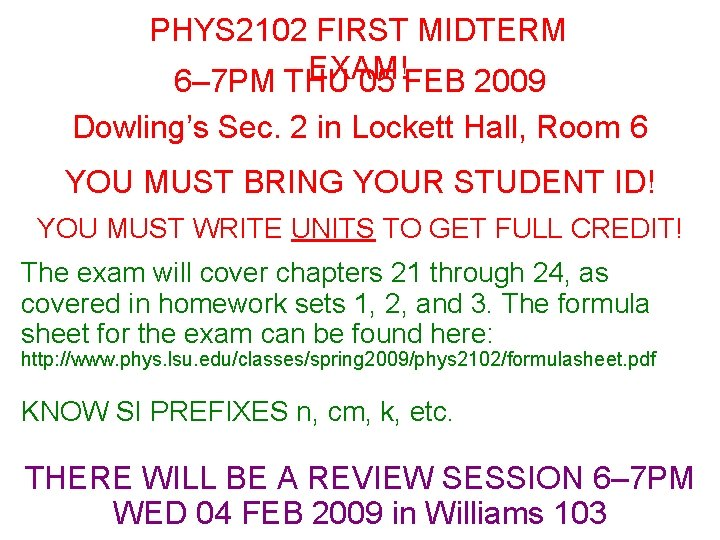PHYS 2102 FIRST MIDTERM EXAM! 6– 7 PM THU 05 FEB 2009 Dowling’s Sec. 2 in Lockett Hall, Room 6 YOU MUST BRING YOUR STUDENT ID! YOU MUST WRITE UNITS TO GET FULL CREDIT! The exam will cover chapters 21 through 24, as covered in homework sets 1, 2, and 3. The formula sheet for the exam can be found here: http: //www. phys. lsu. edu/classes/spring 2009/phys 2102/formulasheet. pdf KNOW SI PREFIXES n, cm, k, etc. THERE WILL BE A REVIEW SESSION 6– 7 PM WED 04 FEB 2009 in Williams 103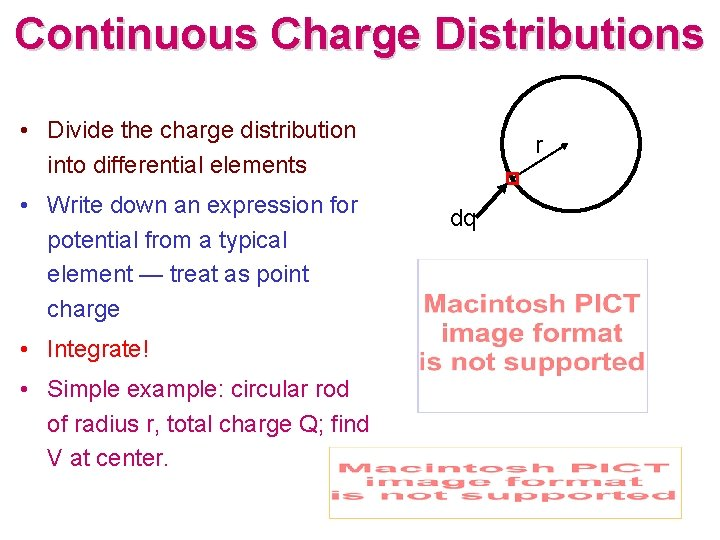Continuous Charge Distributions • Divide the charge distribution into differential elements • Write down an expression for potential from a typical element — treat as point charge • Integrate! • Simple example: circular rod of radius r, total charge Q; find V at center. r dq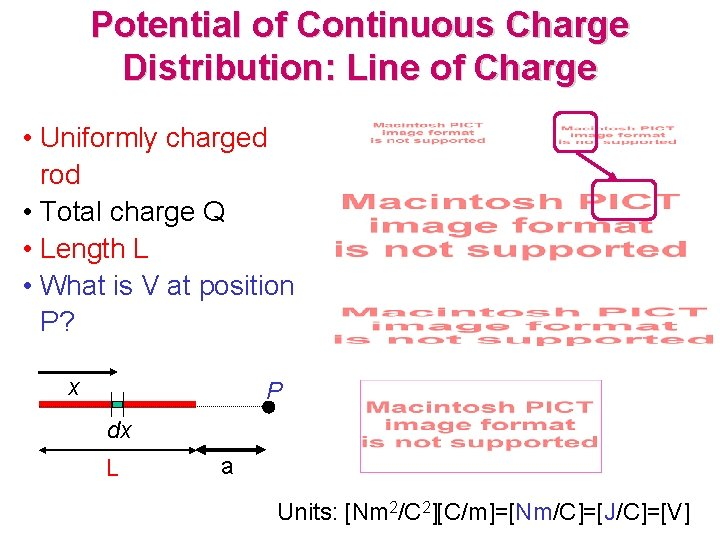Potential of Continuous Charge Distribution: Line of Charge • Uniformly charged rod • Total charge Q • Length L • What is V at position P? x P dx L a Units: [Nm 2/C 2][C/m]=[Nm/C]=[J/C]=[V]Electric Field & Potential: A Simple Relationship! Notice the following: • Point charge: E = k. Q/r 2 V = k. Q/r Focus only on a simple case: electric field that points along +x axis but whose magnitude varies with x. • Dipole (far away): E = kp/r 3 V = kp/r 2 • E is given by a DERIVATIVE of V! • Of course! Note: • MINUS sign! • Units for E: VOLTS/METER (V/m)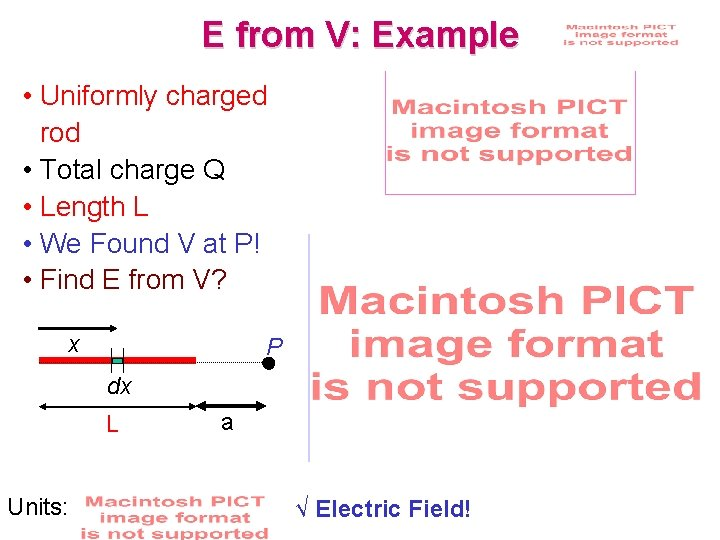E from V: Example • Uniformly charged rod • Total charge Q • Length L • We Found V at P! • Find E from V? x P dx L Units: a √ Electric Field!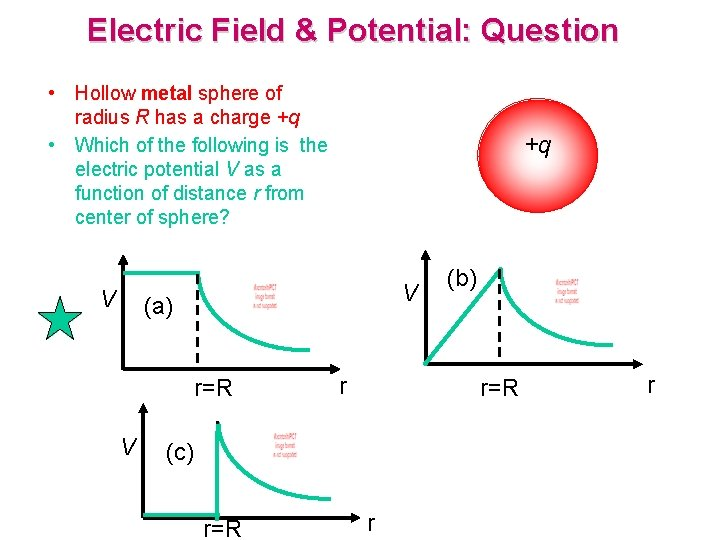Electric Field & Potential: Question • Hollow metal sphere of radius R has a charge +q • Which of the following is the electric potential V as a function of distance r from center of sphere? V +q V (a) r=R V r r=R (c) r=R (b) r rElectric Field & Potential: Example +q E V Outside the sphere: • Replace by point charge! Inside the sphere: • E = 0 (Gauss’ Law) • E = –d. V/dr = 0 IFF V=constant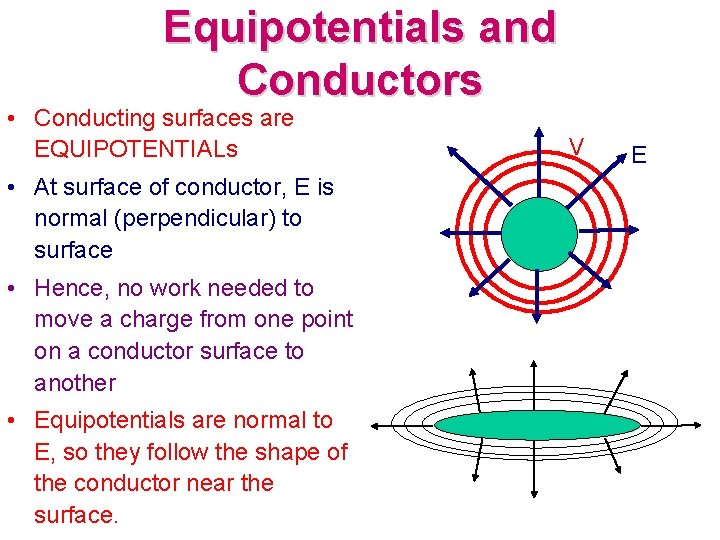Equipotentials and Conductors • Conducting surfaces are EQUIPOTENTIALs • At surface of conductor, E is normal (perpendicular) to surface • Hence, no work needed to move a charge from one point on a conductor surface to another • Equipotentials are normal to E, so they follow the shape of the conductor near the surface. V E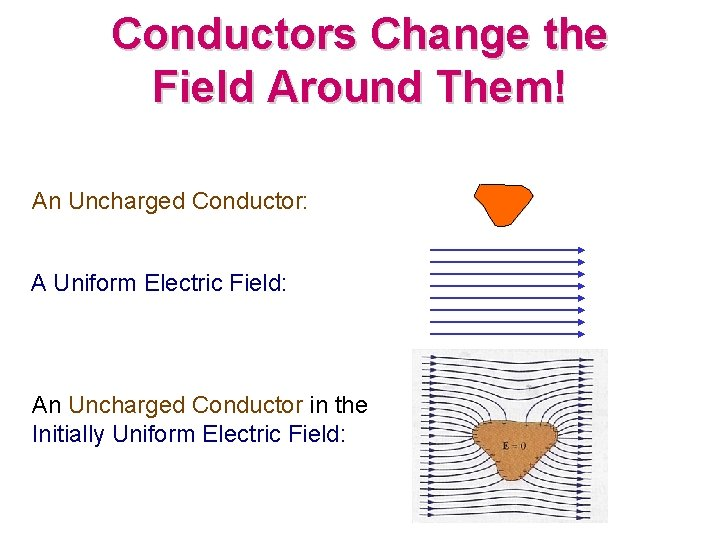Conductors Change the Field Around Them! An Uncharged Conductor: A Uniform Electric Field: An Uncharged Conductor in the Initially Uniform Electric Field:Sharp Conductors • Charge density is higher at conductor surfaces that have small radius of curvature • E = s/e 0 for a conductor, hence STRONGER electric fields at sharply curved surfaces! • Used for attracting or getting rid of charge: – lightning rods – Van de Graaf -- metal brush transfers charge from rubber belt – Mars pathfinder mission -- tungsten points used to get rid of accumulated charge on rover (electric breakdown on Mars occurs at ~100 V/m) (NASA)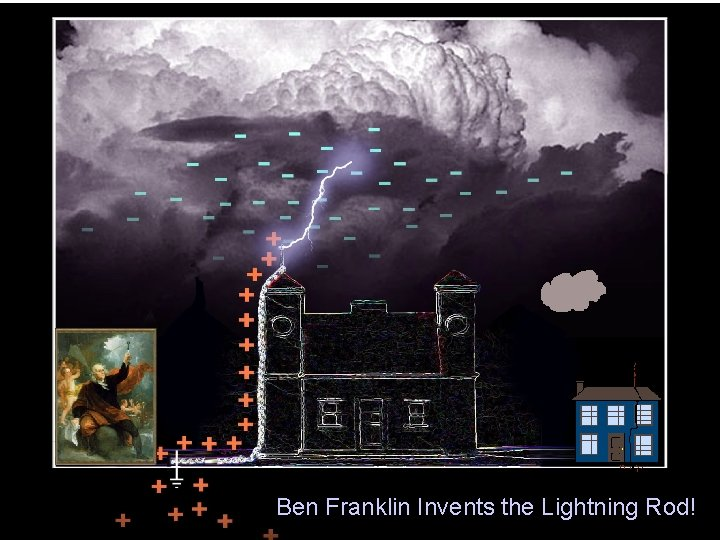Ben Franklin Invents the Lightning Rod!Summary: • Electric potential: work needed to bring +1 C from infinity; units = Volt • Electric potential uniquely defined for every point in space -- independent of path! • Electric potential is a scalar -- add contributions from individual point charges • We calculated the electric potential produced by a single charge: V = kq/r, and by continuous charge distributions : V = kdq/r • Electric field and electric potential: E= -d. V/dx • Electric potential energy: work used to build the system, charge by charge. Use W = U = q. V for each charge. • Conductors: the charges move to make their surface equipotentials. • Charge density and electric field are higher on sharp points of conductors.